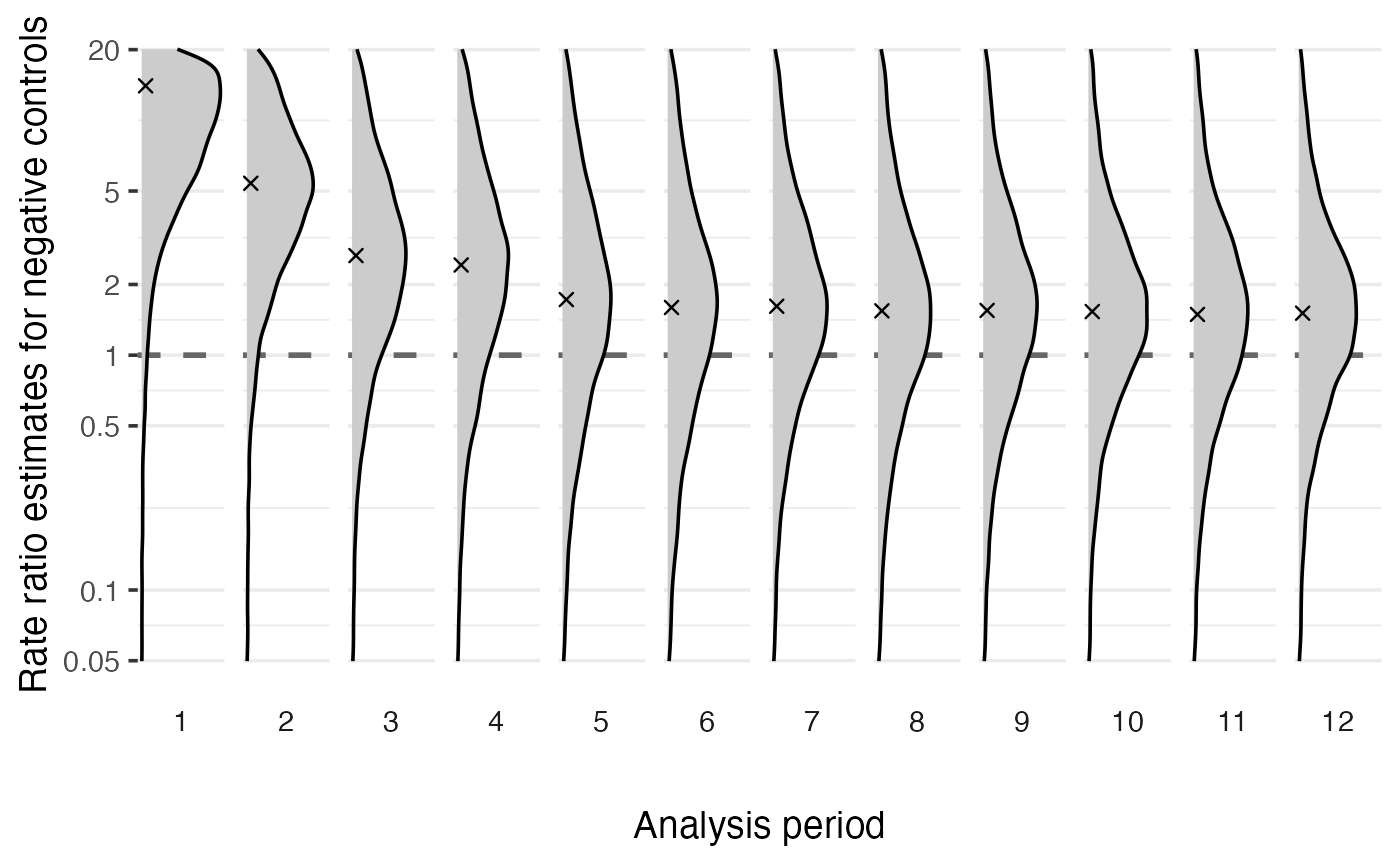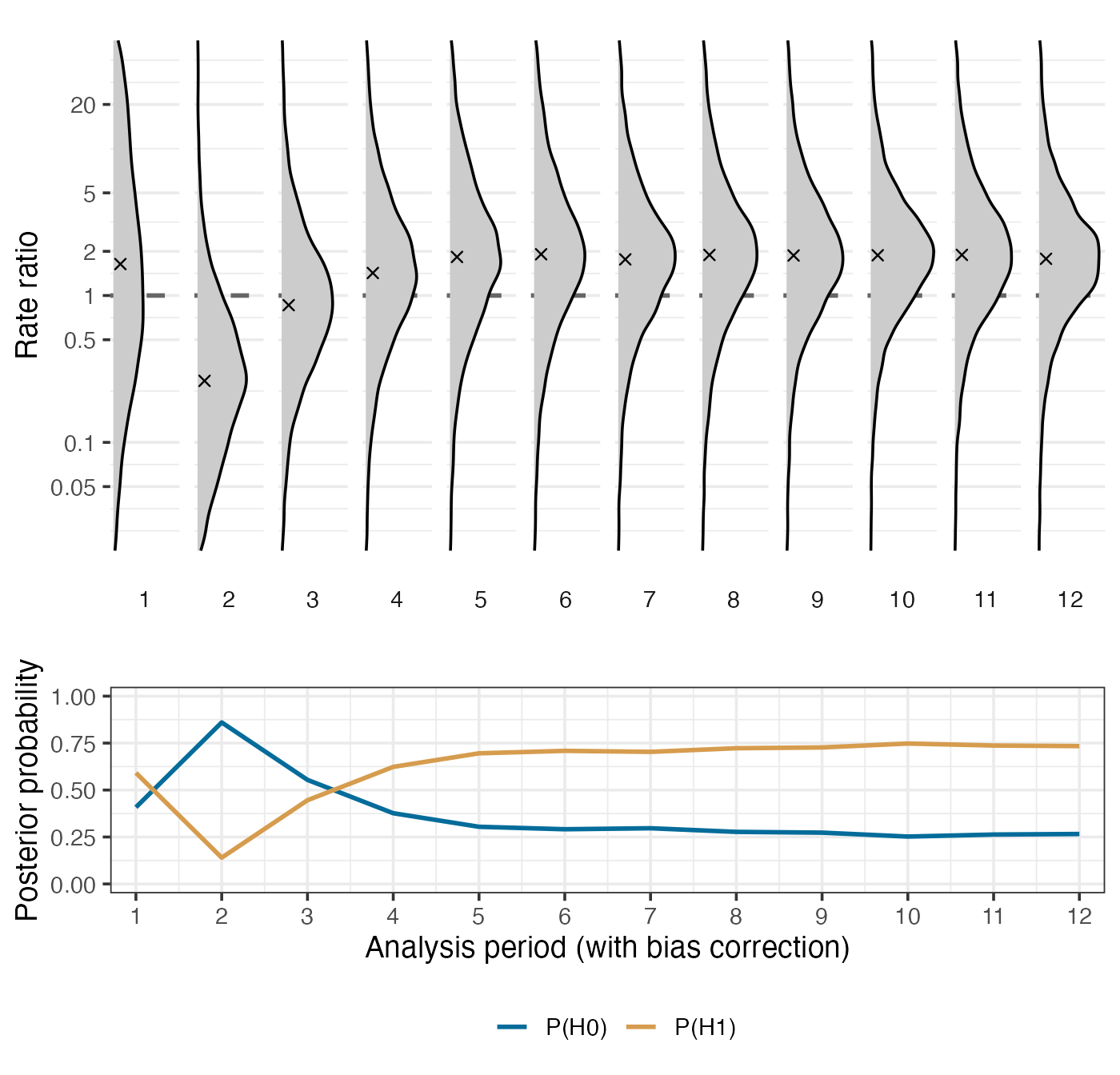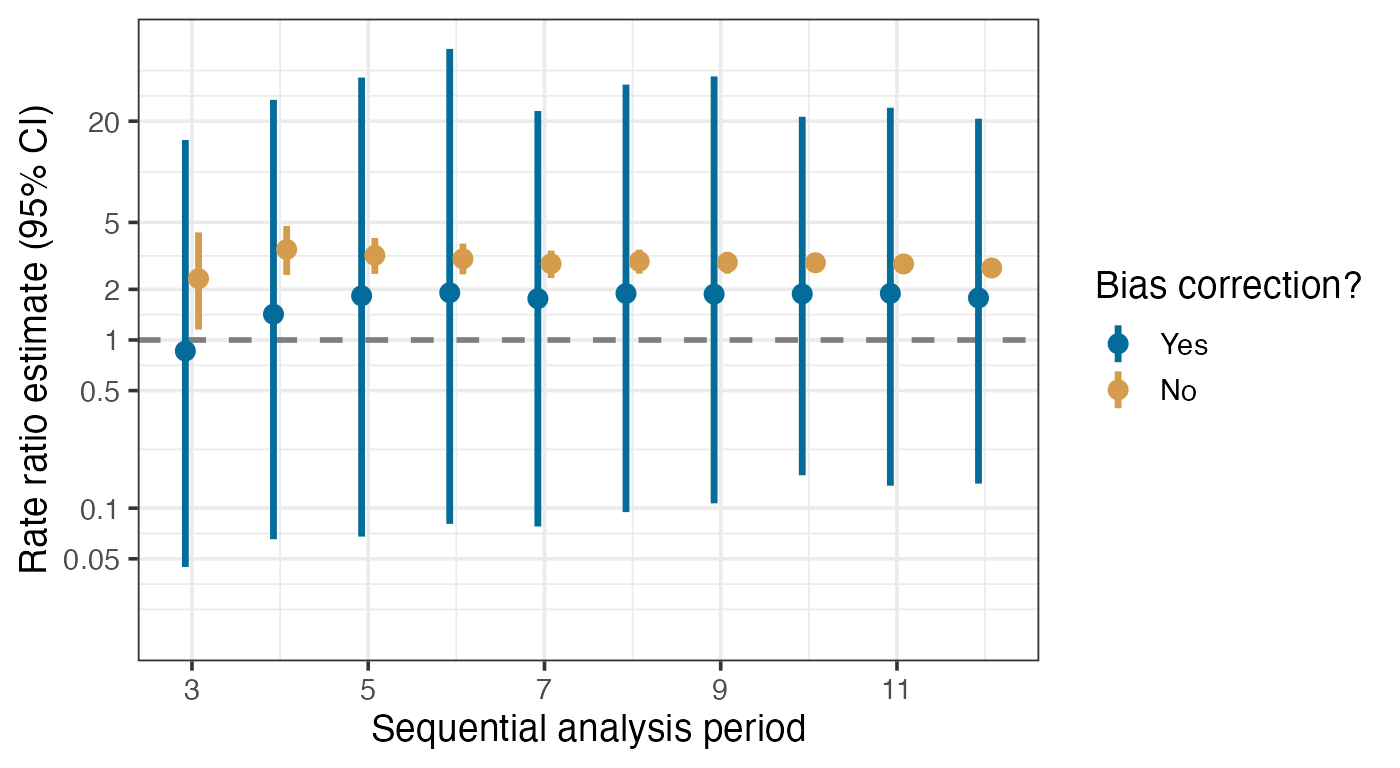## Introduction

We demonstrate Bayesian adaptive bias correction in the context of vaccine safety surveillance using an example dataset. Safety surveillance is a post-approval procedure that monitors vaccine products for adverse events, particularly rare and potentially severe outcomes that may have been undetected in pre-approval clinical trials. It relies on sequential analysis of real-world observational healthcare databases. Therefore, we need to address the challenge of sequential multiple testing as well as residual and unmeasured confounding in observational data.

In EvidenceSynthesis, we have implemented methods of Bayesian adaptive bias correction that can handle sequential analysis and correct for estimation bias induced by the residual confounding in observational health data sources. We perform Bayesian bias correction by jointly modeling the vaccine effect on the adverse event of interest and learning empirical bias distributions using a Bayesian hierarchical modeling approach. The empirical bias distribution arises from simultaneously analyzing a large set of negative control outcomes, which are known to be not associated with the vaccine of interest. Then, using Markov chain Monte Carlo (MCMC), we can obtain the posterior distribution for the de-biased effect size (in terms of the incidence rate ratio, RR) of the vaccine on the outcome of interest.

To accommodate a federated data network setting where patient-level information has to be protected but summary-level information is allowed to be shared, we extract from each data site profile likelihoods regarding the main effect of interest. For each particular pair of vaccine exposure and adverse event outcome, and for each epidemiological design, let $$\mathcal{L}(\beta, \gamma; \mathbf{x})$$ denote the likelihood function. Here, $$\beta$$ is the log RR, the main effect size of interest; $$\gamma$$ represents nuisance parameters; $$\mathbf{x}$$ indicates the data. We profile the multivariate likelihood function and approximate it with a 1d function as follows: $\tilde{\mathcal{L}}(\beta; \mathbf{x}) := \max_{\gamma}\mathcal{L}(\beta, \gamma; \mathbf{x}).$ Then we save the function values evaluated at $$\beta$$ values on an adaptively chosen grid. At each analysis time $$t$$, for a specific vaccine exposure and under a particular design, we would extract a profile likelihood for each negative control outcome and each outcome of interest.

In this vignette, we will go through two key components of bias correction:

• Learn empirical bias distributions by simultaneously analyzing a large set of negative control outcomes, adaptively in time.
• Perform bias correction to analyze a synthesized outcome of interest.

We provide two example data objects, ncLikelihoods and ooiLikelihoods, that contain example profile likelihoods for a large set of negative control outcomes and for a synthetic outcome, respectively. They are extracted from a real-world observational healthcare database, and can be loaded by the commands data("ncLikelihoods") and data("ooiLikelihoods"). Each profile likelihood takes the form of a two-column dataframe, where point includes grid points for $$\beta$$ and value includes the corresponding profile likelihood values.

For example, we can check out the profile likelihood for one of the negative control outcomes at the 1st analysis period:

library(EvidenceSynthesis)

data("ncLikelihoods")
data("ooiLikelihoods")

knitr::kable(ncLikelihoods[][])
point value
-2.30259 -6.90979
-1.79090 -6.39877
-1.27921 -5.88820
-0.76753 -5.37837
-0.25584 -4.86978
0.25584 -4.36326
0.76753 -3.86020
1.27921 -3.36289
1.79090 -2.87519
2.30259 -2.40352

## Learning bias distributions from negative control outcomes

At time $$t$$, given the profile likelihoods regarding the negative control outcomes, we can learn an empirical bias distribution by fitting a hierarchical Bayesian model, using the computeBayesianMetaAnalysis function.

We assume that the estimation biases associated with the negative control outcomes are exchangeable and center around an average’’ bias $$\bar{b}$$ with variability measured by a scale parameter $$\tau$$.

We have implemented a hierarchical normal model and a hierarchical $$t$$-model for the bias distribution, with the normal model as default, and carry out Bayesian posterior inference through a random walk Markov chain Monte Carlo (MCMC).

For instance, to learn the bias distribution at the 1st period using the example dataset, we can call the fitBiasDistribution:

singleBiasDist <- fitBiasDistribution(ncLikelihoods[],
seed = 42
)

The default setting would fit a hierarchical normal model. To fit a more robust distribution that can better handle heavy tails and outliers, we can set robust = TRUE:

singleBiasDistRobust <- fitBiasDistribution(ncLikelihoods[],
robust = TRUE,
seed = 42
)

To learn the bias distribution over multiple analyses, in the setting of either sequential analysis or group analysis, we can call the sequentialFitBiasDistribution function. For example, to adaptively learn all empirical bias distributions across all $$12$$ analysis periods in the example data, under the $$t$$ model:

BiasDistRobust <- sequentialFitBiasDistribution(ncLikelihoods,
robust = TRUE,
seed = 1
)

We can visualize the learned bias distributions over time using the plotBiasDistribution function:

plotBiasDistribution(BiasDistRobust, limits = c(-3, 3))## Perform Bayesian adaptive bias correction

Now we demonstrate how to perform Bayesian adaptive bias correction for sequential analysis. At each analysis time $$t$$, we use the learned empirical bias distribution by analyzing negative controls observed up to time $$t$$ to de-bias our analysis regarding the outcome of interest. We can do this either for time $$t$$ only, or for all analysis timepoints $$t = 1, 2, \ldots, T_{\max}$$.

We provide implementation in the function biasCorrectionInference. To perform bias correction for the $$5$$th analysis, for example:

# select profile likelihoods for the 5th analysis period
ooiLik5 <- list(ooiLikelihoods[["5"]])
ncLik5 <- list(ncLikelihoods[["5"]])

# specify prior mean and prior standard deviation for the effect size (log RR)
bbcResult5 <- biasCorrectionInference(ooiLik5,
ncLikelihoodProfiles = ncLik5,
priorMean = 0,
priorSd = 4,
doCorrection = TRUE,
seed = 42
)

We note that the input should include (1) profile likelihoods on the outcome of interest on the negative controls (e.g., ooiLik5), and (2) profile likelihoods (e.g., ncLik5) or the learned bias distributions. The likelihoods must be provided as a list, where each entry contains the profile likelihood(s) from one analysis period or group. Therefore, if only one period or group of data needs to be analyzed, then the profile likelihoods must be supplied as a list of length $$1$$.

If the bias distribution is already available or has been learned separately, then we can also specify it in the biasDistributions argument. This can be more convenient and efficient if there are more than one outcomes of interest to analyze, as the learned bias distributions can then be re-used. For example:

# learn bias distribution for the 5th analysis period first
biasDist5 <- fitBiasDistribution(ncLikelihoods[["5"]])

# then recycle the bias distribution
bbcResult5 <- biasCorrectionInference(ooiLik5,
biasDistributions = biasDist5,
priorMean = 0,
priorSd = 4,
doCorrection = TRUE,
seed = 42
)

The main output is a summary table of the analysis results, including posterior median and mean (columns median and mean), the 95% credible interval (columns ci95Lb and ci95Ub), and the posterior probability that there is a positive effect (in column p1). Estimates without bias correction can be accessed in the summaryRaw attribute, and so we can compare the results with and without bias correction.

library(dplyr)

knitr::kable(bind_rows(
bbcResult5 %>% mutate(biasCorrection = "yes"),
attr(bbcResult5, "summaryRaw") %>%
mutate(biasCorrection = "no")
) %>%
select(-Id), digits = 4)
median mean ci95Lb ci95Ub p1 biasCorrection
0.5935 0.5939 -0.8838 2.0502 0.7949 yes
1.1588 1.1551 0.9043 1.3981 1.0000 no

To perform sequential analysis with adaptive bias correction over time, we should supply profile likelihoods across all analysis periods/groups in the input. For example, if we use the already learned bias distributions BiasDistRobust:

bbcSequential <- biasCorrectionInference(ooiLikelihoods,
biasDistributions = BiasDistRobust,
priorMean = 0,
priorSd = 4,
doCorrection = TRUE,
seed = 42
)

We can then check out the sequential analysis results:

knitr::kable(bbcSequential %>% select(period = Id, median:p1), digits = 4)
period median mean ci95Lb ci95Ub p1
1 0.4875 0.7422 -3.5436 5.8970 0.5856
2 -1.3418 -1.3949 -4.3864 1.5127 0.1428
3 -0.1727 -0.1850 -3.0050 2.7624 0.4346
4 0.3537 0.3656 -2.6551 3.4021 0.6258
5 0.6284 0.6229 -2.4261 3.9465 0.7042
6 0.6528 0.6457 -2.4722 3.9950 0.7099
7 0.5284 0.5207 -2.3062 3.4768 0.6894
8 0.6556 0.6423 -2.2494 3.4861 0.7305
9 0.6126 0.6111 -2.1726 3.5031 0.7179
10 0.6316 0.6464 -2.1094 3.3503 0.7420
11 0.6685 0.6763 -1.8358 3.6872 0.7465
12 0.5929 0.5964 -1.8419 3.1823 0.7377

The plotBiasCorrectionInference function provides some simple plotting functionality to visualize the analysis results. For example, we can visualize the posterior distributions of the bias corrected log RR across analysis periods, along with posterior probabilities for a positive effect size (P(H1)) or a non-positive effect size (P(H0)):

plotBiasCorrectionInference(bbcSequential,
type = "corrected",
limits = c(-4, 4)
)Meanwhile, we can also compare the estimates with and without bias correction. It is also possible to only examine certain analysis periods/groups by specifying the ids argument.

plotBiasCorrectionInference(bbcSequential,
type = "compare",
limits = c(-4, 4),
ids = as.character(3:12)
)